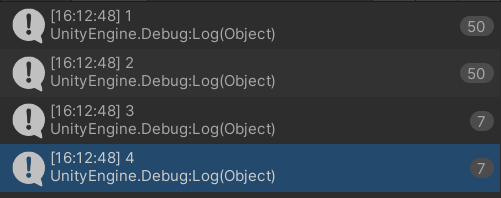# Coroutine Sequence not calling functions every time.

I am making a script that for each room (in a dungeon), creates a room in all four directions, relative to the new room. The room that is created then checks if there is space to spawn, if not it shrinks and if still doesn’t fit the function ends.

To check if there are any other rooms colliding, i wait after each room to create before creating the next one. For that i made a IEnumerator function which is called on start().

``````private IEnumerator CreateNewRooms()
{
float newWidth;
float newLenght;

newWidth = Random.Range(4, 30);
newLenght = Random.Range(4, 30);
Debug.Log("1");
yield return StartCoroutine(DungeonGenerator.Instance.CreateRoom(newWidth, newLenght, position + Vector3.left * (width + newWidth) * 0.5f));

newWidth = Random.Range(5, 30);
newLenght = Random.Range(5, 30);
Debug.Log("2");
yield return StartCoroutine(DungeonGenerator.Instance.CreateRoom(newWidth, newLenght, position + Vector3.right * (width + newWidth) * 0.5f));

newWidth = Random.Range(5, 30);
newLenght = Random.Range(5, 30);
Debug.Log("3");
yield return StartCoroutine(DungeonGenerator.Instance.CreateRoom(newWidth, newLenght, position + Vector3.back * (lenght + newLenght) * 0.5f));

newWidth = Random.Range(5, 30);
newLenght = Random.Range(5, 30);
Debug.Log("4");
yield return StartCoroutine(DungeonGenerator.Instance.CreateRoom(newWidth, newLenght, position + Vector3.forward * (lenght + newLenght) * 0.5f));
}
``````

Before each function i Debug a number to check if the function works. I found that only function “1” and “2” are called enough times (The max amount of times a room can be spawned is 50).Why does this happen?

EDIT:
Here is the code from DungeonGenerator.cs:

``````public IEnumerator CreateRoom(float width, float lenght, Vector3 position)
{
bool isValid = false;
Collider[] hitColliders = Physics.OverlapBox(
new Vector3(position.x, position.y - 3, position.z), // Position
new Vector3(width * 0.495f, 6, lenght * 0.495f), // Size
Quaternion.identity // Rotation
);

if (index < iterations)
{
while (!isValid)
{
if (
hitColliders.Length <= 0
) { isValid = true; break; }

else
{
width--;
lenght--;
}

if (width < 5 || lenght < 5)
{
yield return null;
}
}

if (isValid)
{
// Creates room gameobject
GameObject room = new GameObject("Room");

trigger.isTrigger = true;
trigger.size = new Vector3(width, 4, lenght);
trigger.center = new Vector3(0, 2, 0);

// Sets room variables
room.GetComponent<Room>().width = width;
room.GetComponent<Room>().lenght = lenght;
room.GetComponent<Room>().position = position;

room.GetComponent<Room>().floorMat = floorMat;
room.GetComponent<Room>().wallMat = wallMat;

index++;
}
}

}
``````

I haven’t run your code first hand so apologies if I am reading the code wrong, but the problem I see is within the While loop in the CreateRoom method. The only break condition in the While loop is when the room becomes valid. But if it never becomes valid, you’re just constantly reducing the width/length and then waiting until the next frame. I suspect you have an infinite loop and don’t even realise because it’s inside a coroutine thus isn’t freezing the game.

You need to fix the logic so it correctly breaks out of the While loop, rather than waiting until next frame.

As for the use of Coroutines… you could probably do away with them entirely and use normal void methods. Unless the physics engine is a limitation and doesn’t update until the next frame, and therefore you’re waiting a frame out of necessity for the collision? In which case you could simply add a ‘yield return null’ statement after each CreateRoom call in the CreateNewRooms method.

I hope this helps, my answer is untested.